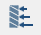# Hydrostatic Pressure

Define pressure exerted by a fluid at equilibrium.

Hydrostatic pressure is defined as a pressure exerted by a fluid at equilibrium at a given point within the fluid, due to the force of gravity. Hydrostatic pressure increases in proportion to depth measured from the surface because of the increasing weight of fluid exerting downward force from above.

1. In the Project Tree, open the Analysis Workbench.
2. On the Analysis Workbench, click the(Hydrostatic pressure) icon.
3. In the modeling window, select faces to apply hydrostatic pressure to.
4. Optional: You can select the Add tangent faces check box.
5. Locate the fluid surface by defining the X, Y, and Z coordinates under Coordinates of a point at liquid surface.
Tip: You can also locate the coordinates in the modeling window using the graphical representation of the hydrostatic pressure load. Drag the ball around the model until it is in the desired position.
6. Define the Depth direction X, Y, and Z directions.
7. Specify units and value for Liquid density.
8. Specify units and value for Pressure at liquid surface.
9. Click OK.
The hydrostatic pressure load appears in the Project Tree. If selected, a graphic representation appears on the model.
Note: If a gravity direction is already defined, the depth direction uses this existing definition. In this case, you can locate the surfaces elevation, but not change its direction.